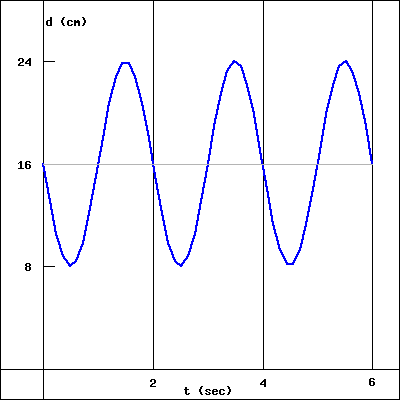A weight is suspended from the ceiling by a spring. Let $d$ be the distance in centimeters from the ceiling to the weight. When the weight is motionless, $d = 16 \, \mbox{cm}$. If the weight is disturbed, it begins to bob up and down, or oscillate. Then $d$ is a periodic function of $t$, the time in seconds, so $d = f(t)$. Consider the graph of $d = f(t)$ below, which represents the distance of the weight from the ceiling at time $t$.(a) Based on the graph of $d =f(t)$ above, which of the statements below correctly describes the motion of the weight as it bobs up and down?

(b) How long does it take the weight to bounce completely up and down (or down and up) and return to its starting position?
(e) What is the amplitude of the graph of $d = f(t)$?